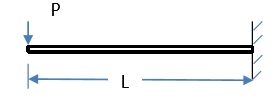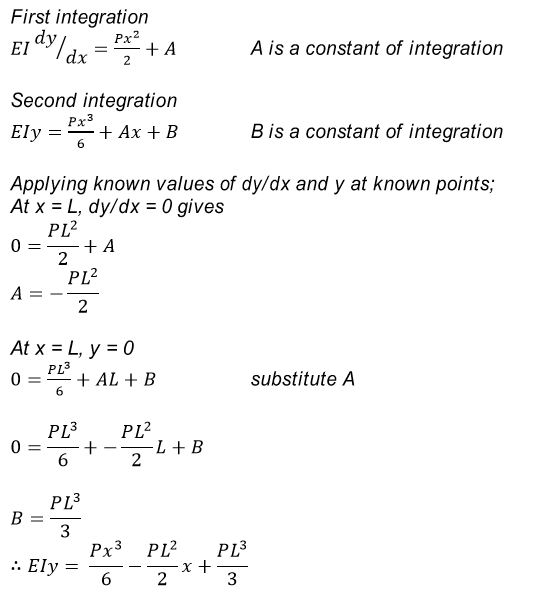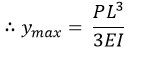##### Question

In: Mechanical Engineering

# DEFLECTION OF BEAMS

Determine the maximum deflection on the Cantilever beam with a concentrated load p at the free end as shown## Solutions

##### Expert Solution

Where E = Young’s modulus of beam material, I = the second moment of area of the beam cross-section taken about neutral axis, y = deflection of the beam at a point a distance x, and Mx = bending moment at a point a distance x. The equation is called the Euler-Bernoulli equation. The deflection can be obtained by integrating the moment equation twice. Two integration constants will result. The constants are found by considering slope dy/dx or deflection y at known points.

Deflection and slope at known points

1. For built-in end; deflection y = 0, slope dy/dx = 0

2. For simple support; at the support, deflection =0 but slope dy/dx is not equal to zero.The maximum deflection occurs at the free end, x = 0.